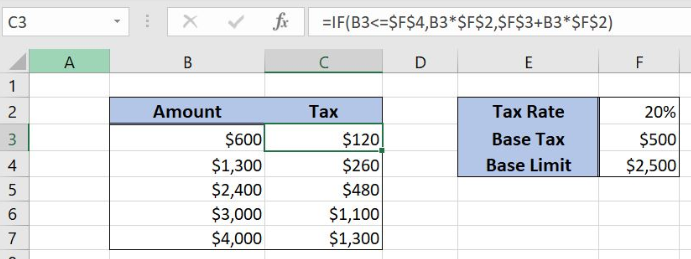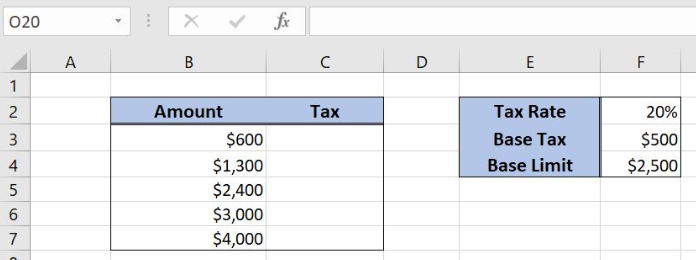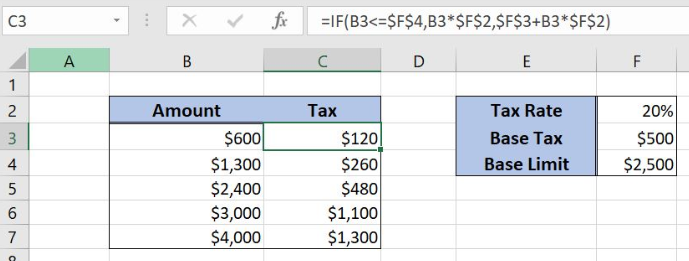Get instant live expert help with Excel or Google Sheets“My Excelchat expert helped me in less than 20 minutes, saving me what would have been 5 hours of work!”

#### Post your problem and you’ll get Expert help in seconds.

Your message must be at least 40 characters
Our professional Expert are available now. Your privacy is guaranteed.

# Tax rate calculation with fixed base

Excel allows a user to calculate a tax rate with fixed base using the IF function. This step by step tutorial will assist all levels of Excel users in calculating a tax rate with fixed base.Figure 1. The result of the IF function

## Syntax of the IF Formula

The generic formula for the IF function is:

`=IF(logical_test, value_if_true, value_if_false)`

The parameters of the IF function are:

• logical_test – a logical expression that we want to check
• value_if_true – a value which the function returns if a logical_test is TRUE
• value_if_false – a value which the function returns if a logical_test is FALSE.

## Setting up Our Data for the IF Formula

Let’s first look at the data that we will use in the example. Our table consists of 2 columns: “Amount” (column B) and “Tax” (column C). The Tax rate is in the cell F2, the base tax in F3 and the base limit in F4. In column C, we want to get a tax for the amount.Figure 2. Data that we will use in the IF example

## Using the IF Function to Calculate a Tax Rate with Fixed Base

If the amount is less than the base limit in F4 (\$2,500), then the tax is 20% of the amount (B3). If the amount is greater than the tax is the base tax from F3 (\$500) plus 20% of the amount (B3). The result in the cell C3.

The formula is:

`=IF(B3<=\$F\$4, B3*\$F\$2, \$F\$3+B3*\$F\$2)`

The logical_test is B3<=\$F\$4. The value_if_true is B3*\$F\$2, while the value_if_false is \$F\$3+B3*\$F\$2. In the cell C3, we want to get the result. Please note that we have to fix F2, F3 and F4, as these cells are not changing when the formula is copied down the cells.

To apply the IF function, we need to follow these steps:

• Select cell C3 and click on it
• Insert the formula: `=IF(B3<=\$F\$4, B3*\$F\$2, \$F\$3+B3*\$F\$2)`
• Press enter
• Drag the formula down to the other cells in the column by clicking and dragging the little “+” icon at the bottom-right of the cell.Figure 3. Using the IF function to calculate a tax rate with fixed base

Since the \$600 is less than \$2,500, the logical condition is true, so the tax is 20% of \$600, which is \$120 in C3.

In the last row, the amount is greater than \$2,500 (\$4,000), so the logical condition is false. Therefore the tax is \$500 + 20% of \$4,000. The result is \$1,300 in the cell C7.

Most of the time, the problem you will need to solve will be more complex than a simple application of a formula or function. If you want to save hours of research and frustration, try our live Excelchat service! Our Excel Experts are available 24/7 to answer any Excel question you may have. We guarantee a connection within 30 seconds and a customized solution within 20 minutes.

### Did this post not answer your question? Get a solution from connecting with the expert.Another blog reader asked this question today on Excelchat:## Subscribe to Excelchat.coAnother blog reader asked this question today on Excelchat: